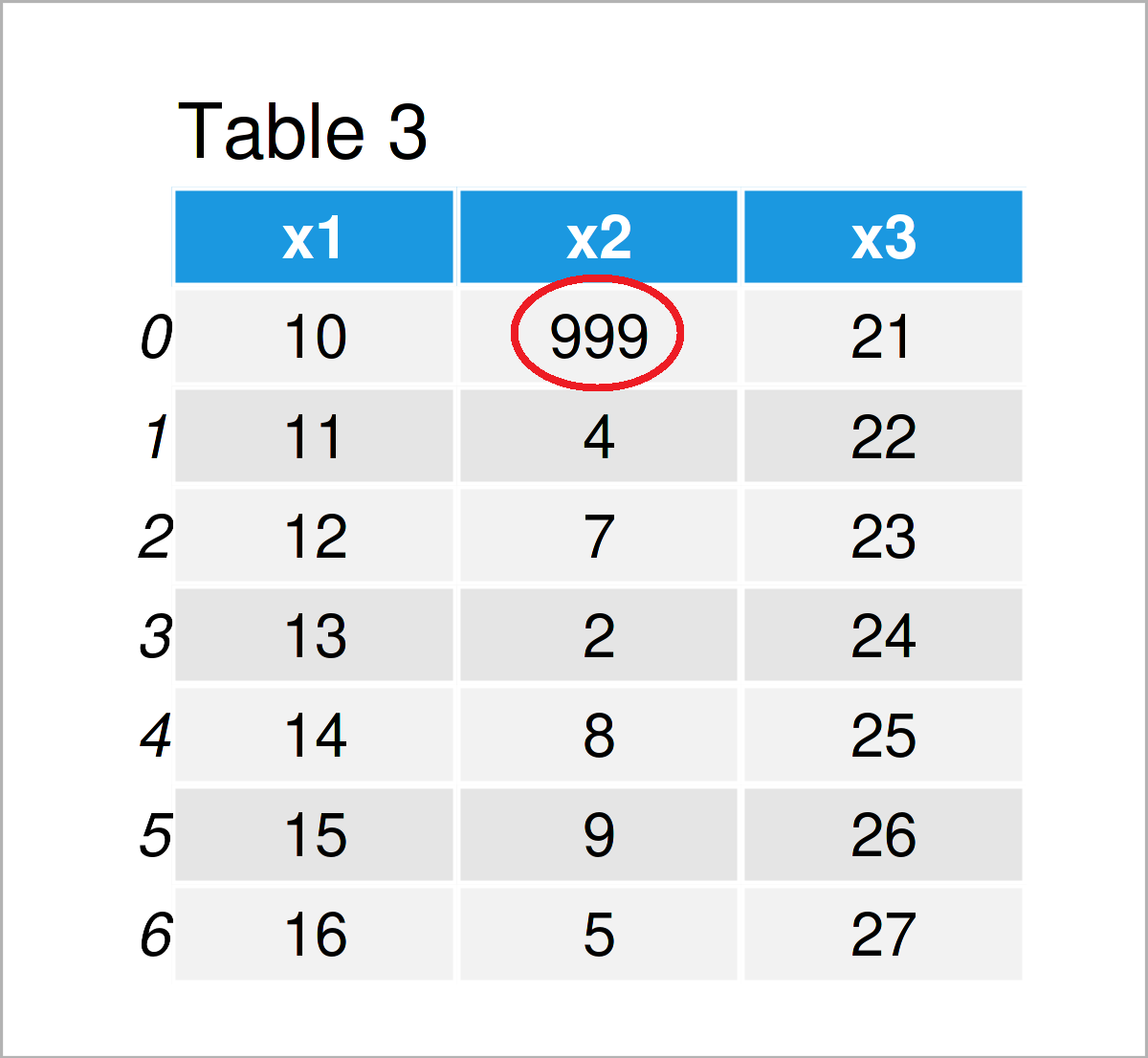# Check If Two pandas DataFrames are Equal in Python (Example) | equals() Function Explained

In this article, I’ll show how to test whether two pandas DataFrames contain exactly the same values in the Python programming language.

The article contains the following content blocks:

You’re here for the answer, so let’s get straight to the Python code:

## Exemplifying Data & Libraries

First, we have to import the pandas library:

`import pandas as pd                          # Import pandas library in Python`

The following three pandas DataFrames are used as a basis for this Python programming language tutorial:

```data1 = pd.DataFrame({'x1':range(10, 17),    # Create first pandas DataFrame
'x2':[3, 4, 7, 2, 8, 9, 5],
'x3':range(21, 28)})
print(data1)                                 # Print first pandas DataFrame```As you can see based on Table 1, our first example data is a DataFrame comprising seven rows and three columns called x1, x2, and x3.

Let’s create another data set:

```data2 = pd.DataFrame({'x1':range(10, 17),    # Create second pandas DataFrame
'x2':[3, 4, 7, 2, 8, 9, 5],
'x3':range(21, 28)})
print(data2)                                 # Print second pandas DataFrame```In Table 2 it is shown that we have created another pandas DataFrame containing exactly the same values as our first data set (more on that later).

One more:

```data3 = pd.DataFrame({'x1':range(10, 17),    # Create third pandas DataFrame
'x2':[999, 4, 7, 2, 8, 9, 5],
'x3':range(21, 28)})
print(data3)                                 # Print third pandas DataFrame```In Table 3 you can see that we have created a third pandas DataFrame with the previously shown code. This DataFrame contains a different value in the first row and the second column.

Let’s compare these data set systematically!

## Example: Test If Two pandas DataFrames are the Same Using equals() Function

In this example, I’ll illustrate how to check whether two pandas DataFrames are identical.

For this task, we can apply the equals function of the pandas library.

The following Python syntax compare our first and second DataFrames:

```print(data1.equals(data2))                   # Compare data1 & data2
# True```

After executing the previous Python code, the logical indicator True has been returned, i.e. the data sets data1 and data2 are identical.

Let’s apply the equals function once again to our first and third data sets:

```print(data1.equals(data3))                   # Compare data1 & data3
# False```

This time, the Python console has returned the logical indicator False, i.e. the two pandas DataFrames data1 and data3 are not equal.

## Video, Further Resources & Summary

If you need further info on the Python codes of this article, I recommend watching the following video on my YouTube channel. In the video, I’m explaining the Python code of this article in a live session.

Please accept YouTube cookies to play this video. By accepting you will be accessing content from YouTube, a service provided by an external third party.If you accept this notice, your choice will be saved and the page will refresh.

In addition to the video, you might read the related tutorials on this website. I have released several articles already.

Summary: You have learned in this post how to check whether two pandas DataFrames are duplicate copies and consist of identical values in Python programming. Tell me about it in the comments section, in case you have additional questions.

Subscribe to the Statistics Globe Newsletter